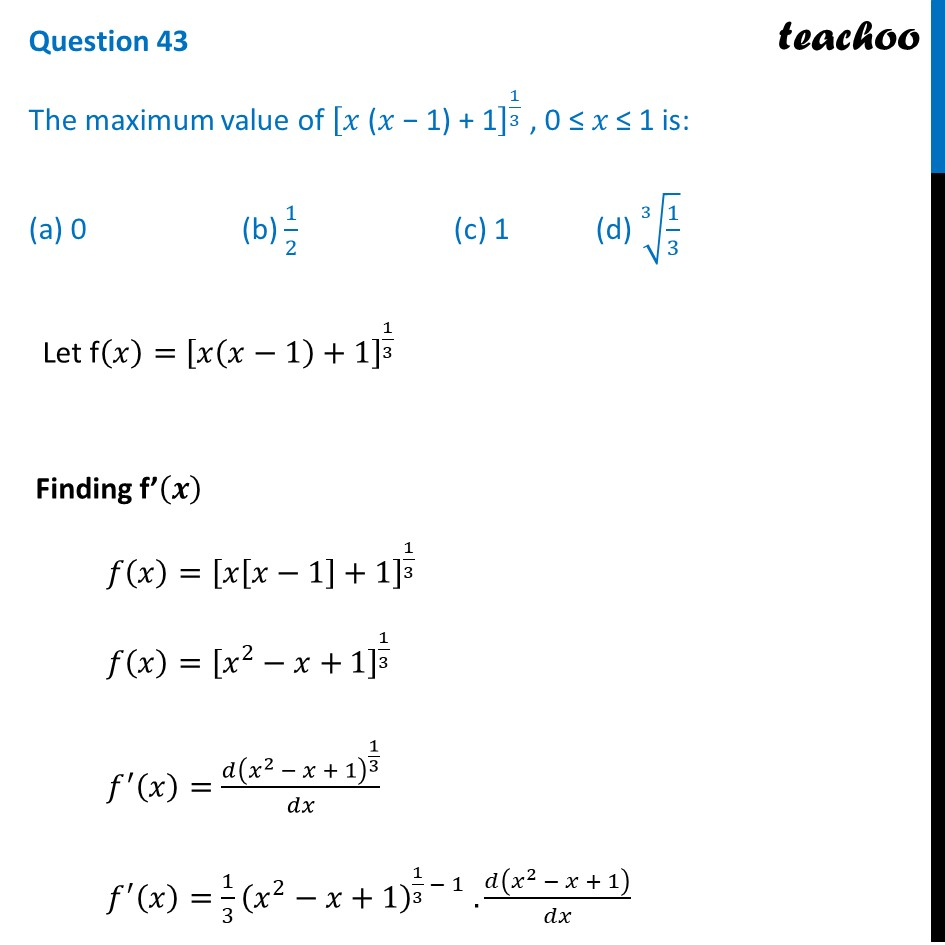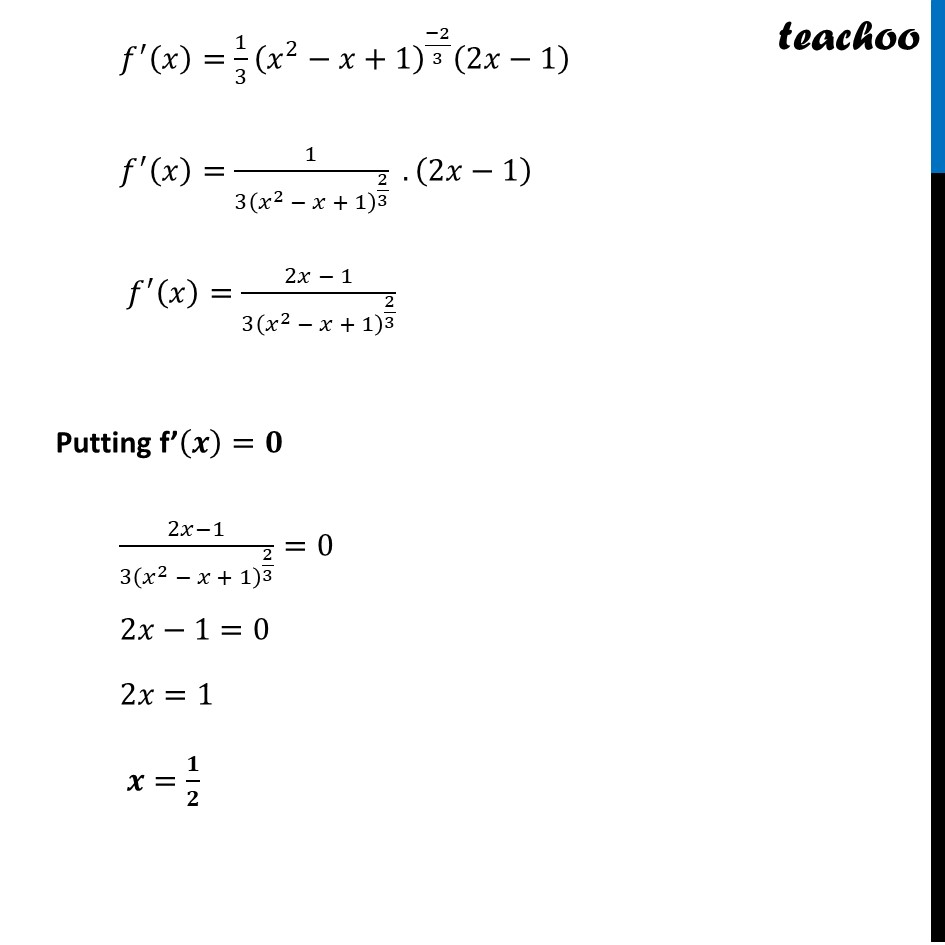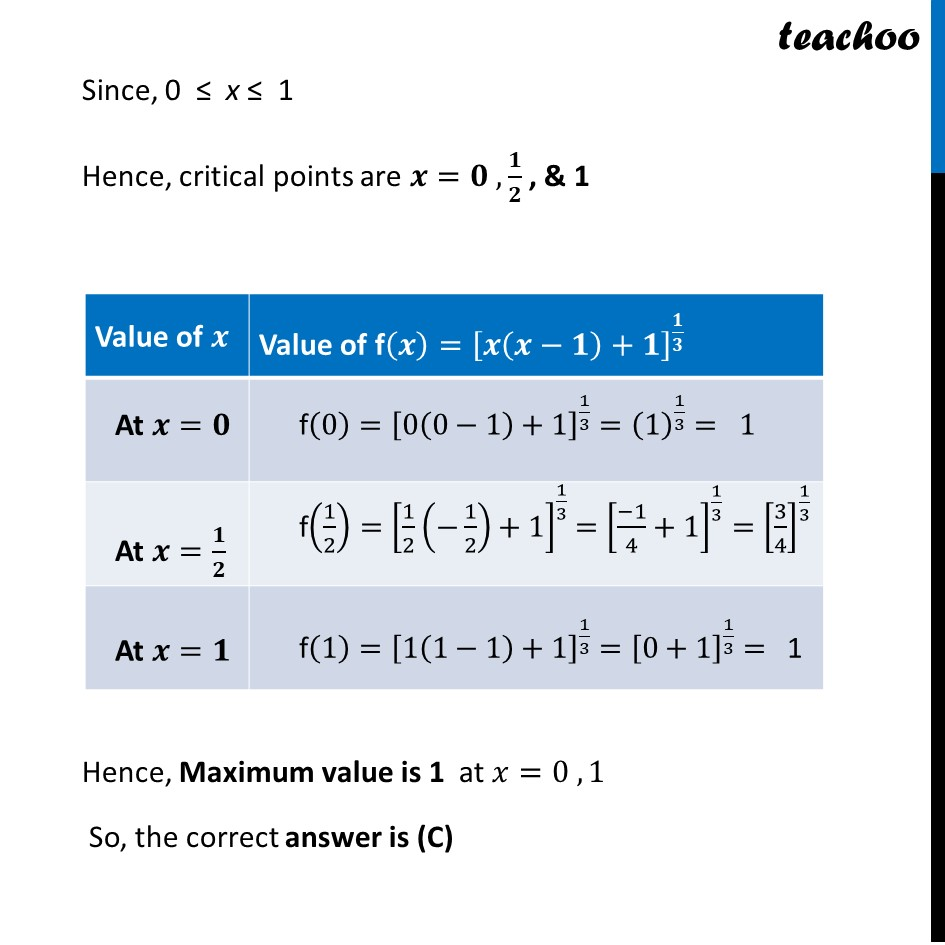CBSE Class 12 Sample Paper for 2022 Boards (MCQ Based - for Term 1)

Class 12
Solutions of Sample Papers and Past Year Papers - for Class 12 Boards

## (a) 0       (b) 1/2         (c) 1     (d) ∛(1/3)

This question is inspired from Ex 6.5,29 (MCQ) - Chapter 6 Class 12 - Application of DerivativesGet live Maths 1-on-1 Classs - Class 6 to 12

### Transcript

Question 43 The maximum value of ["𝑥 (𝑥 − 1) + 1" ]^(1/3) , 0 ≤ 𝑥 ≤ 1 is: (a) 0 (b) 1/2 (c) 1 (d) ∛(1/3) Let f(𝑥)=[𝑥(𝑥−1)+1]^(1/3) Finding f’(𝒙) 𝑓(𝑥)=[𝑥[𝑥−1]+1]^(1/3) 𝑓(𝑥)=[𝑥^2−𝑥+1]^(1/3) 𝑓^′ (𝑥)=(𝑑(𝑥^2 − 𝑥 + 1)^(1/3))/𝑑𝑥 𝑓^′ (𝑥)=1/3 (𝑥^2−𝑥+1)^(1/3 − 1) . 𝑑(𝑥^2 − 𝑥 + 1)/𝑑𝑥 𝑓^′ (𝑥)=1/3 (𝑥^2−𝑥+1)^((−2)/3) (2𝑥−1) 𝑓^′ (𝑥)=1/(3(𝑥^2 − 𝑥 + 1)^(2/3) ) .(2𝑥−1) 𝑓^′ (𝑥)=(2𝑥 − 1)/(3(𝑥^2 − 𝑥 + 1)^(2/3) ) Putting f’(𝒙)=𝟎 (2𝑥−1)/(3(𝑥^2 − 𝑥 + 1)^(2/3) )=0 2𝑥−1=0 2𝑥=1 𝒙=𝟏/𝟐 Since, 0 ≤ x ≤ 1 Hence, critical points are 𝒙=𝟎 ,𝟏/𝟐 , & 1 Hence, Maximum value is 1 at 𝑥=0 , 1 So, the correct answer is (C)